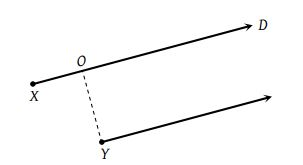Two point sources X and Y emit waves of same frequency and speed but Y lags in phase behind X by 2πl radian. If there is a maximum in direction D the distance XO using n as an integer is given by(1) $\frac{\lambda }{2}\left(n-l\right)$

(2) $\lambda \left(n+l\right)$

(3) $\frac{\lambda }{2}\left(n+l\right)$

(4) $\lambda \left(n-l\right)$

Explanation is a part of a Paid Course. To view Explanation Please buy the course.

Difficulty Level: